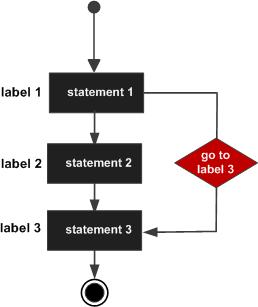### Forum

Wiki## Pascal - Goto Statement

Contents[Hide]

A goto statement in Pascal provides an unconditional jump from the goto to a labeled statement in the same function.

NOTE: Use of goto statement is highly discouraged in any programming language because it makes difficult to trace the control flow of a program, making the program hard to understand and hard to modify. Any program that uses a goto can be rewritten so that it doesn't need the goto.

## 1. Syntax:

The syntax for a goto statement in Pascal is as follows:

```goto label;
...
...
label: statement;
```

Here label must be an unsigned integer label whose value can be from 1 to 9999.

## 2. Flow Diagram:## 3. Example:

The following program illustrates the concept.

```program exGoto;
label 1;
var
a : integer;
begin
a :=10;
(* repeat until loop execution *)
1: repeat
if( a =15 )then
begin
(* skip the iteration *)
a := a + 1;
goto 1;
end;
writeln('value of a: ', a);
a:= a + 1;
until a = 20;
end.
```

When the above code is compiled and executed, it produces following result:

```value of a: 10
value of a: 11
value of a: 12
value of a: 13
value of a: 14
value of a: 16
value of a: 17
value of a: 18
value of a: 19

```